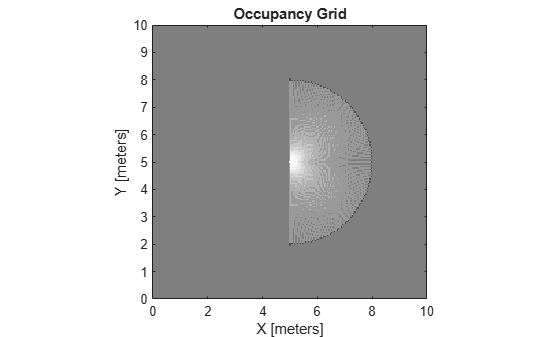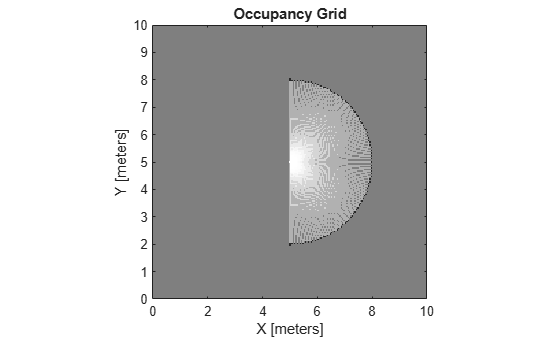occupancyMap

Create occupancy map with probabilistic values

Description

occupancyMap creates a 2-D occupancy grid map object. Each cell in the occupancy grid has a value representing the probability of the occupancy of that cell. Values close to 1 represent a high probability that the cell contains an obstacle. Values close to 0 represent a high probability that the cell is not occupied and obstacle free.

Occupancy maps are used in navigation algorithms such as path planning (see plannerRRT). They are also used in mapping applications for finding collision-free paths, performing collision avoidance, and calculating localization (see monteCarloLocalization). You can modify your occupancy map to fit your specific application.

The occupancyMap objects support local coordinates, world coordinates, and grid indices. The first grid location with index (1,1) begins in the top-left corner of the grid.

Use the occupancyMap class to create 2-D maps of an environment with probability values representing different obstacles in your world. You can specify exact probability values of cells or include observations from sensors such as laser scanners.

Probability values are stored using a binary Bayes filter to estimate the occupancy of each grid cell. A log-odds representation is used, with values stored as int16 to reduce the map storage size and allow for real-time applications.

Creation

Description

map = occupancyMap(width,height) creates a 2-D occupancy map object representing a world space of width and height in meters. The default grid resolution is 1 cell per meter.

example

map = occupancyMap(width,height,resolution) creates an occupancy map with a specified grid resolution in cells per meter. resolution sets the Resolution property.

map = occupancyMap(rows,cols,resolution,'grid') creates an occupancy map with the specified number of rows and columns and with the resolution in cells per meter. The values of rows and cols sets the GridSize property.

map = occupancyMap(p) creates an occupancy map from the values in matrix p. The grid size matches the size of the matrix, with each cell probability value interpreted from the matrix location.

example

map = occupancyMap(p,resolution) creates an occupancy map from the specified matrix and resolution in cells per meter.

map = occupancyMap(sourcemap) creates an object using values from another occupancyMap object.

map = occupancyMap(sourcemap,resolution) creates an object using values from another occupancyMap object, but resamples the matrix to have the specified resolution.

Input Arguments

expand all

Map width, specified as a scalar in meters.

Map height, specified as a scalar in meters.

Grid resolution, specified as a scalar in cells per meter.

Number of rows in grid, specified as a positive scalar integer.

Number of columns in grid, specified as a positive scalar integer.

Input occupancy grid, specified as a matrix of probability values from 0 to 1. The size of the grid matches the size of the matrix. Each matrix element corresponds to the probability of the grid cell location being occupied. Values close to 1 represent a high certainty that the cell contains an obstacle. Values close to 0 represent certainty that the cell is not occupied and obstacle free.

Occupancy map object, specified as a occupancyMap object.

Properties

expand all

Threshold below which cells are considered obstacle-free, specified as a scalar between 0 and 1 inclusive. Cells with probability values below this threshold are considered obstacle free. This property also defines the free locations for path planning when using objects like plannerRRT.

Data Types: double

Threshold above which cells are considered occupied, specified as a scalar. Cells with probability values above this threshold are considered occupied.

Data Types: double

Saturation limits for probability, specified as a 1-by-2 real-valued vector representing the minimum and maximum values, in that order. Values above or below these saturation values are set to the minimum and maximum values. This property reduces oversaturating of cells when incorporating multiple observations.

Data Types: double

Number of rows and columns in grid, stored as a 1-by-2 real-valued vector representing the number of rows and columns, in that order.

Data Types: double

Grid resolution, stored as a scalar in cells per meter representing the number and size of grid locations.

Data Types: double

Minimum and maximum values of x-coordinates in local frame, stored as a two-element horizontal vector of the form [min max]. Local frame is defined by LocalOriginInWorld property.

Data Types: double

Minimum and maximum values of y-coordinates in local frame, stored as a two-element horizontal vector of the form [min max]. Local frame is defined by LocalOriginInWorld property.

Data Types: double

Minimum and maximum world range values of x-coordinates, stored as a 1-by-2 vector representing the minimum and maximum values, in that order.

Data Types: double

Minimum and maximum world range values of y-coordinates, stored as a 1-by-2 vector representing the minimum and maximum values, in that order.

Data Types: double

[x,y] world coordinates of the bottom-left corner of the grid, specified as a 1-by-2 vector.

Data Types: double

Location of the origin of the local frame in world coordinates, specified as a two-element vector, [xLocal yLocal]. Use the move function to shift the local frame as your vehicle moves.

Data Types: double

Location of the bottom-left corner of the grid in local coordinates, specified as a two-element vector, [xLocal yLocal].

Data Types: double

Default value for unspecified map locations including areas outside the map, specified as a scalar between 0 and 1 inclusive.

Data Types: double

Object Functions

 checkOccupancy Check locations for free, occupied, or unknown values copy Create copy of occupancy grid getOccupancy Get occupancy value of locations grid2local Convert grid indices to local coordinates grid2world Convert grid indices to world coordinates inflate Inflate each occupied grid location insertRay Insert ray from laser scan observation local2grid Convert local coordinates to grid indices local2world Convert local coordinates to world coordinates move Move map in world frame occupancyMatrix Convert occupancy grid to double matrix raycast Compute cell indices along a ray rayIntersection Find intersection points of rays and occupied map cells setOccupancy Set occupancy value of locations show Show grid values in a figure syncWith Sync map with overlapping map updateOccupancy Integrate probability observations at locations world2grid Convert world coordinates to grid indices world2local Convert world coordinates to local coordinates

Examples

collapse all

Create an empty occupancy grid map.

map = occupancyMap(10,10,20);

Input pose of the vehicle, ranges, angles, and the maximum range of the laser scan.

pose = [5,5,0];
ranges = 3*ones(100,1);
angles = linspace(-pi/2,pi/2,100);
maxrange = 20;

Create a lidarScan object with the specified ranges and angles.

scan = lidarScan(ranges,angles);

Insert the laser scan data into the occupancy map.

insertRay(map,pose,scan,maxrange);

Show the map to see the results of inserting the laser scan.

show(map)Check the occupancy of the spot directly in front of the vehicle.

getOccupancy(map,[8 5])
ans = 0.7000

Add a second reading and view the update to the occupancy values. The additional reading increases the confidence in the readings. The free and occupied values become more distinct.

insertRay(map,pose,scan,maxrange);
show(map)getOccupancy(map,[8 5])
ans = 0.8448

Convert a portable graymap (PGM) file containing a ROS map into an occupancyMap for use in MATLAB.

Import the image using imread. Crop the image to the playpen area.

imageCropped = image(750:1250,750:1250);
imshow(imageCropped)PGM values are expressed from 0 to 255 as uint8. Normalize these values by converting the cropped image to double and dividing each cell by 255. This image shows obstacles as values close to 0. Subtract the normalized image from 1 to get occupancy values with 1 representing occupied space.

imageNorm = double(imageCropped)/255;
imageOccupancy = 1 - imageNorm;

Create the occupancyMap object using an adjusted map image. The imported map resolution is 20 cells per meter.

map = occupancyMap(imageOccupancy,20);
show(map)Limitations

Occupancy values have a limited resolution of ±0.001. The values are stored as int16 using a log-odds representation. This data type limits resolution, but saves memory when storing large maps in MATLAB®. When calling setOccupancy and then getOccupancy, the value returned might not equal the value you set. For more information, see the log-odds representations section in Occupancy Grids.

If memory size is a limitation, consider using binaryOccupancyMap instead. The binary occupancy map uses less memory with binary values, but still works with Navigation Toolbox™ algorithms and other applications.